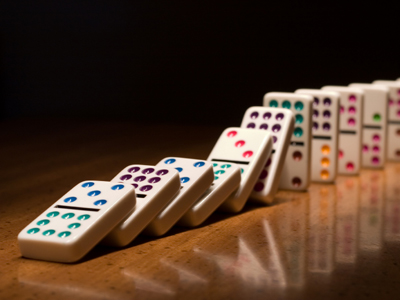There are 28 dominoes in a set.

# Verbal Reasoning - Complete the Sum 2

This Math quiz is called 'Verbal Reasoning - Complete the Sum 2' and it has been written by teachers to help you if you are studying the subject at elementary school. Playing educational quizzes is an enjoyable way to learn if you are in the 3rd, 4th or 5th grade - aged 8 to 11.

It costs only \$12.50 per month to play this quiz and over 3,500 others that help you with your school work. You can subscribe on the page at Join Us

In this quiz you have to find the number that will complete the sum correctly. The only operations that will be used are multiply, divide, add and subtract.

Sometimes you have to work out the sum to find the last number, and sometimes you have to find a number in the middle of the sum. The more you practice these sums, the better your mental arithmetic will be.

Example:

26 + 7 = 18 + ?

13
14
15
16

This is because 26 + 7 = 33. 18 + ? = 33. This makes 15 the only possible answer.
1.
Find the number that will complete the sum below correctly.

? + 6 = 30 ÷ 3
4
5
15
40
This is because 30 ÷ 3 = 10, and 4 + 6 = 10. Only 4 works for this sum. 30 is the number of sides in a triacontagon in geometry, and 30 years of marriage results in the 'Pearl Anniversary'
2.
Find the number that will complete the sum below correctly.

14 ÷ 7 = 30 - ?
26
24
28
22
This is because 14 ÷ 7 = 2, and 30 - 28 = 2. Only 28 works. 28 is a number which has many interesting features: it is the product of 7 and 4, it is the product of 14 and 2, and it is also the number of teeth in a normal set of adult teeth, and also corresponds to the number of dominoes in a set!
3.
Find the number that will complete the sum below correctly.

22 + ? = 11 + 112
110
121
111
101
This is because 11 + 112 = 123. To get to 123 from 22 we need to add 101. Simple!
4.
Find the number that will complete the sum below correctly.

44 - 8 = ? x 9
6
5
4
3
This is because 44 - 8 = 36, which is 9 x 4. Only 4 works
5.
Find the number that will complete the sum below correctly.

4 x 3 = ? - 17
19
49
39
29
This is because 4 x 3 is twelve, and 29 - 17 is twelve. Only 29 works
6.
Find the number that will complete the sum below correctly.

712 ÷ 2 = 178 x ?
12
22
2
21
This is because 712 ÷ 2 = 356, which is 178 x 2
7.
Find the number that will complete the sum below correctly.

? ÷ 6 = 36
226
236
216
246
This is because 216 ÷ 6 = 36. Just as well you learned your 36 times tables!
8.
Find the number that will complete the sum below correctly.

32 ÷ 4 = ? + 4
0.4
40
400
4
This is because 32 ÷ 4 = 8. 4 + 4 = 8
9.
Find the number that will complete the sum below correctly.

12 x 4 = ? + 39
3
9
8
4
This is because 12 x 4 = 48. Something plus 39 = 48 makes 9 the only possible answer. 39 is a very interesting number, being made up of the product of two prime numbers, (3 and 13) and also the number in the novel The 39 Steps, by John Buchan, which was made even more famous in the film by Alfred Hitchcock
10.
Find the number that will complete the sum below correctly.

117 x 3 = 300 + ?
15
14
51
56
This is because 117 x 3 = 351. 300 + 51 = 351
Author:  Stephen O'Hara